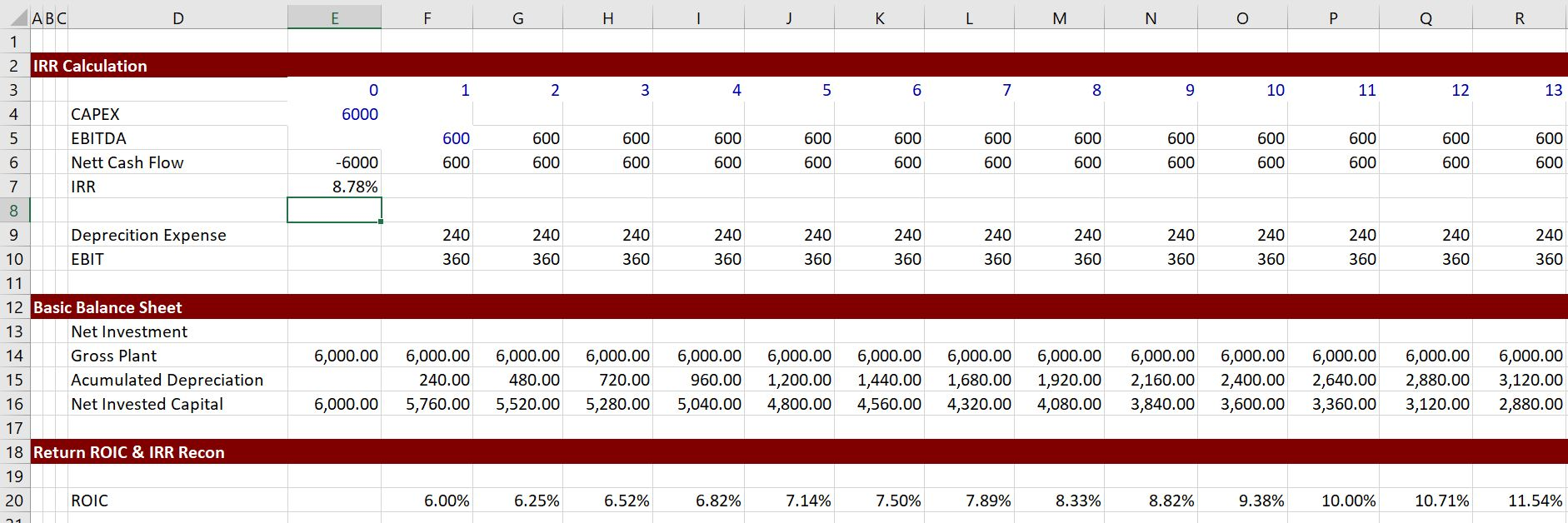# Reconciliation of IRR on Single Investments with ROI for the Corporation

The relationship between ROI and IRR can be confusing.  If investors focus on ROI (or ROIC or ROE) but managers focus on IRR (or project IRR or Equity IRR), what is the relationship between IRR and ROI.  In the short-term for single investments the IRR and ROI are different.  But in the long-run under certain extreme conditions the ROI and the IRR can be the same.  This is only when the discount rate used in the reconciliation process is the IRR itself.

Economic evaluation of a new investment generally involves assessing the IRR on the investment. But management generally is concerned with presenting ROIC and ROE which are key measures of relative performance. The question addressed on below is whether the return on investment and the IRR converge in the long run and whether a company that continually makes investments that earn a given IRR will converge to the same ROI.

The video and files below show that if the IRR itself is used as the discount rate, then the ROI is a weighted average of ROI over the life of an investment. But,the ROI is only the same as the IRR in each year if a form of economic depreciation is used. More importantly, the ROI is lower than the ROI in early years of the investment and higher in later years. This means that if a corporation has recently made a large investment, a low ROE is expected. On the other hand, if investments are old then the ROI will be higher than the IRR.  By pressing the button below you can download the file that works through IRR and ROI reconciliation issues.

## Simple IRR and ROI Reconciliation

The screen shot below demonstrates the issue for a simple case with no taxes and no debt.  In this case the IRR on the project from a capital investment is 8.78% but the ROI begins at 6% and then increases to above the IRR of 8.67%.  The reason for the difference is simply depreciation expense. (The IRR and ROI can be reconciled with economic depreciation which is rarely applied).When the net invested capital is discounted at the IRR itself and the discounted value of the investment is used as a weighting factor for computing a weighted average ROIC, then the weighted average ROIC is equal to the IRR as shown in the screen shot below.  (The weighted average ROIC is computed using the SUMPRODUCT function).IF a different discount rate than the IRR is used, the weighted average ROIC will be different from the IRR.  When the discount rate is below the IRR the weighted average ROIC is higher.  When the discount rate is above the IRR the weighted average ROIC is lower.

## IRR and ROI Reconciliation with a Portfolio of Investments

The video below works through cases where multiple investments are made and evaluates the resulting corporate ROI. In general the ROIC is close to the IRR in the long-run, although the ROIC converges to a higher level. The file that goes along with this video is the one you can download by pressing the button above.

## MIRR is BS

Some people think they are fancy using the MIRR instead of the IRR.  They can plop some kind of WACC coming from magic potion into the MIRR calculation and think they have solved the distortions created by the re-investment assumption in the IRR.  This may be true for some bond applications.  It is not at all true for corporate or project finance.  For example if the IRR is higher than the WACC, the MIRR turns out to be lower than the IRR.  Indeed, all the MIRR does is to push the IRR towards the discount rate that you input.

It is better to compute the weighted average ROIC and use a weighting that reflects the cost of capital. This ROIC is the driver of investment value.